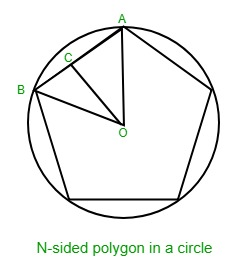# Side of a regular n-sided polygon circumscribed in a circle

Given two integers r and n where n is the number of sides of a regular polygon and r is the radius of the circle this polygon is circumscribed in. The task is to find the length of the side of polygon.Examples:

Input: n = 5, r = 11
Output: 12.9256

Input: n = 3, r = 5
Output: 8.6576

## Recommended: Please try your approach on {IDE} first, before moving on to the solution.

Approach: Consider the image above and let angle AOB be theta then theta = 360 / n.
In right angled triangle, angle ACO = 90 degrees and angle AOC = theta / 2.
So, AC = OA * sin(theta / 2) = r * sin(theta / 2)
Therefore, side of the polygon, AB = 2 * AC i.e. 2 * r * sin(theta / 2).

Below is the implementation of the above approach:

## C++

 `// C++ implementation of the approach ` `#include ` `using` `namespace` `std; ` ` `  `// Function to calculate the side of the polygon ` `// circumscribed in a circle ` `float` `calculateSide(``float` `n, ``float` `r) ` `{ ` `    ``float` `theta, theta_in_radians; ` ` `  `    ``theta = 360 / n; ` `    ``theta_in_radians = theta * 3.14 / 180; ` ` `  `    ``return` `2 * r * ``sin``(theta_in_radians / 2); ` `} ` ` `  `// Driver Code ` `int` `main() ` `{ ` ` `  `    ``// Total sides of the polygon ` `    ``float` `n = 3; ` ` `  `    ``// Radius of the circumscribing circle ` `    ``float` `r = 5; ` ` `  `    ``cout << calculateSide(n, r); ` `} `

## Java

 `// Java  implementation of the approach ` `import` `java.lang.Math; ` `import` `java.io.*; ` ` `  `class` `GFG { ` `     `  `// Function to calculate the side of the polygon ` `// circumscribed in a circle ` `static` `double` `calculateSide(``double`  `n, ``double` `r) ` `{ ` `    ``double` `theta, theta_in_radians; ` ` `  `    ``theta = ``360` `/ n; ` `    ``theta_in_radians = theta * ``3.14` `/ ``180``; ` ` `  `    ``return` `2` `* r * Math.sin(theta_in_radians / ``2``); ` `} ` ` `  `// Driver Code ` `    ``public` `static` `void` `main (String[] args) { ` ` `  `    ``// Total sides of the polygon ` `    ``double` `n = ``3``; ` ` `  `    ``// Radius of the circumscribing circle ` `    ``double` `r = ``5``; ` `    ``System.out.println (calculateSide(n, r)); ` `    ``} ` `//This code is contributed by akt_mit     ` `} `

## Python3

 `# Python 3 implementation of the approach ` `from` `math ``import` `sin ` ` `  `# Function to calculate the side of  ` `# the polygon circumscribed in a circle ` `def` `calculateSide(n, r): ` `    ``theta ``=` `360` `/` `n ` `    ``theta_in_radians ``=` `theta ``*` `3.14` `/` `180` ` `  `    ``return` `2` `*` `r ``*` `sin(theta_in_radians ``/` `2``) ` ` `  `# Driver Code ` `if` `__name__ ``=``=` `'__main__'``: ` `     `  `    ``# Total sides of the polygon ` `    ``n ``=` `3` ` `  `    ``# Radius of the circumscribing circle ` `    ``r ``=` `5` ` `  `    ``print``(``'{0:.5}'``.``format``(calculateSide(n, r))) ` ` `  `# This code is contributed by ` `# Sanjit_Prasad `

## C#

 `// C# implementation of the approach  ` ` `  `using` `System; ` ` `  `class` `GFG {  ` `         `  `    ``// Function to calculate the side of the polygon  ` `    ``// circumscribed in a circle  ` `    ``static` `double` `calculateSide(``double` `n, ``double` `r)  ` `    ``{  ` `        ``double` `theta, theta_in_radians;  ` `     `  `        ``theta = 360 / n;  ` `        ``theta_in_radians = theta * 3.14 / 180;  ` `     `  `        ``return` `Math.Round(2 * r * Math.Sin(theta_in_radians / 2),4);  ` `    ``}  ` ` `  `        ``// Driver Code  ` `    ``public` `static` `void` `Main () {  ` ` `  `    ``// Total sides of the polygon  ` `    ``double` `n = 3;  ` ` `  `    ``// Radius of the circumscribing circle  ` `    ``double` `r = 5;  ` `     `  `    ``Console.WriteLine(calculateSide(n, r));  ` `    ``}  ` `    ``// This code is contributed by Ryuga ` `}  `

## PHP

 ` `

Output:

```8.6576
```

My Personal Notes arrow_drop_upCheck out this Author's contributed articles.

If you like GeeksforGeeks and would like to contribute, you can also write an article using contribute.geeksforgeeks.org or mail your article to contribute@geeksforgeeks.org. See your article appearing on the GeeksforGeeks main page and help other Geeks.

Please Improve this article if you find anything incorrect by clicking on the "Improve Article" button below.

Article Tags :
Practice Tags :

Be the First to upvote.

Please write to us at contribute@geeksforgeeks.org to report any issue with the above content.